# How to write a real number(ℝ) symbol in LaTeX?

Set of real number is represented by the ℝ symbol. For this, you need to pass the argument R in \mathbb command in latex.

Symbol Real numbers
Type Set symbol
Package asmmath,… etc
Command \mathbb{R}
Example \mathbb{R} → ℝ

In latex, this \mathbb command is located in more than one package. So, you look at this table below

 amsfonts \mathbb{R} →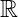amssymb \mathbb{R} →txfonts \mathbb{R} →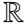pxfonts \mathbb{R} →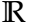Real number symbol structure is the same for amsfonts and amssymb packages but slightly different for txfonts and pxfonts packages.

\documentclass{article}
\usepackage{amsfonts}
\begin{document}
$a,b\in\mathbb{R}$
\end{document}

Output :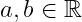## Real part from complex number in LaTeX

A complex number is made up of two parts, real and complex. And the real part is identified by the ℜ(z) symbol. By default, the \Re(z) command is used to define this ℜ(z) symbol

\documentclass{article}
\begin{document}
$z=a+ib$
$\Re(z) = a$
\end{document}

Output :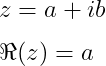You can denote real part symbols using more different methods instead of the default method in latex. For example

1. Using a physics package that contains \Re command to denote the real part. And \Re command return Re(z) symbol instead of (z) symbol.

\documentclass{article}
\usepackage{physics}
\begin{document}
$z = a+ib$
$\Re(z) = a$
\end{document}

Output :2. Secondly, you use the amsmath package. And re syntax must be passed into the \operatorname command.

\documentclass{article}
\usepackage{amsmath}
\begin{document}
$z = a+ib$
$\operatorname{Re}(z) = a$
\end{document}

Output :3. Thirdly being amsfonts can be used to present the \mathfrak command. And you can pass both R and Re as arguments in this \mathfrak command.

\documentclass{article}
\usepackage{amsfonts}
\begin{document}
$z = a+ib$
$\mathfrak{R}(z) = a$
$\mathfrak{Re}(z) = a$
\end{document}

Output :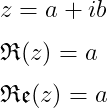If you look at the above outputs, you will understand that it is best to use Re(z) instead of (z) to denote the real part symbol. And for this, using the physics package and \Re command is the best practice.#### Md Jidan Mondal

LaTeX expert with over 10 years of experience in document preparation and typesetting. Specializes in creating professional documents, reports, and presentations using LaTeX.